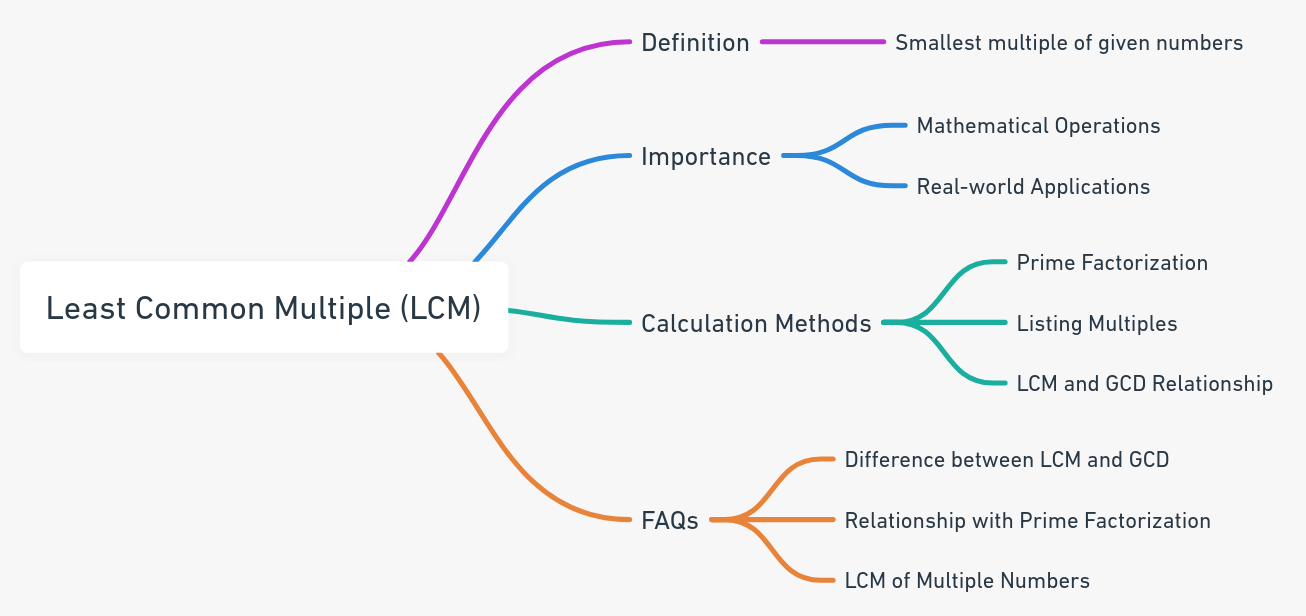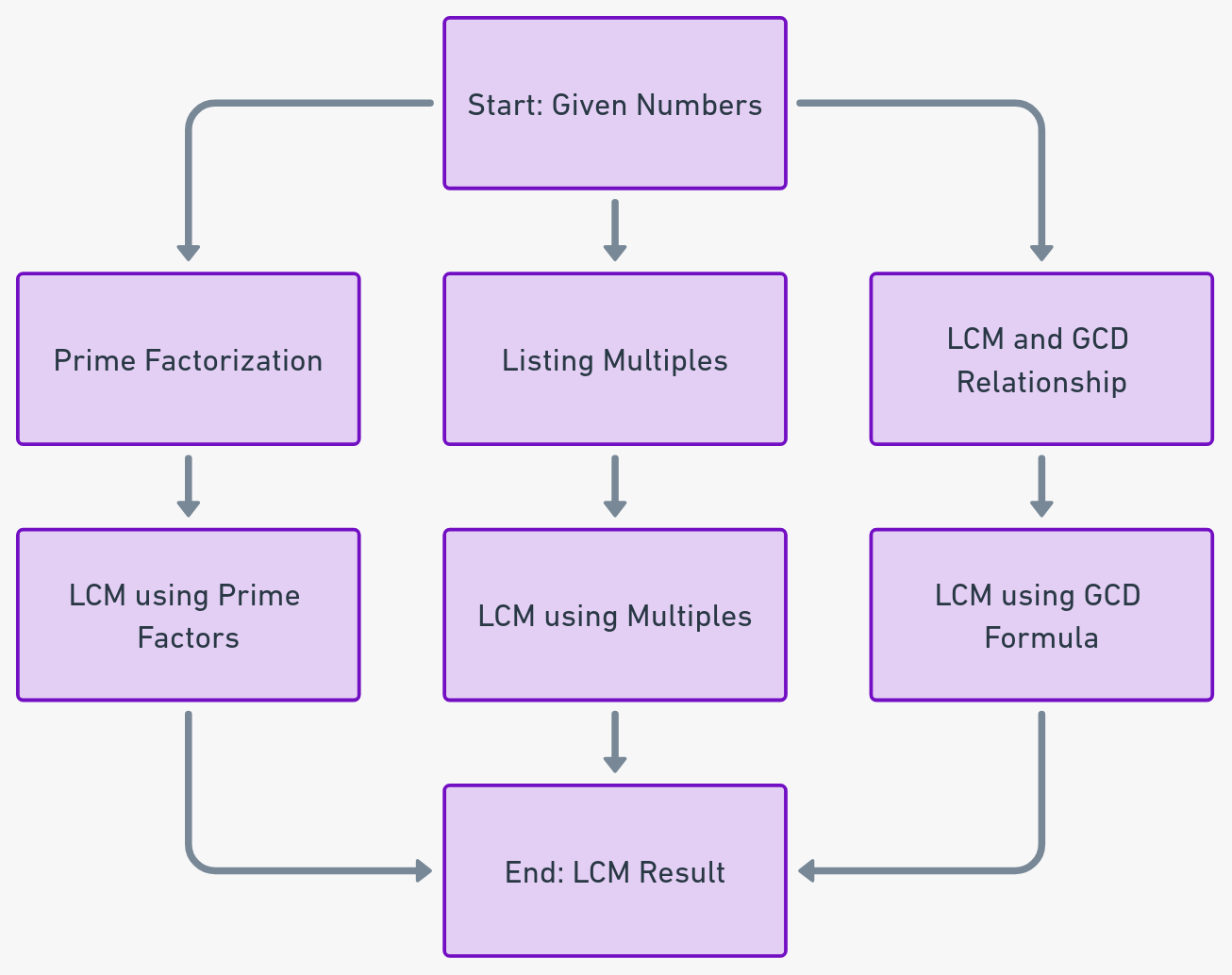Even The mini Tools Can Empower People to Do Great Things.

# Least Common Multiple (LCM) Calculator: From Basics to Advanced Understanding

Discover the Least Common Multiple (LCM) with our user-friendly calculator. Understand the concept, its significance, and how prime factorization plays a role. Dive deep into the world of LCM.

Least Common Multiple Calculator

To find the least common multiple, enter all the positive integers separated by comma [example1] or space [example2]:

Embed Least Common Multiple Calculator Widget

## About Least Common Multiple CalculatorThe Least Common Multiple Calculator is used to find out the least common multiple (LCM) of two or more positive integers. It also provides a step-by-step proof using the prime factorization method, ensuring transparency and understanding.

## What is the Least Common Multiple?

The least common multiple, often abbreviated as LCM, is the smallest number that is a multiple of two or more given numbers. It plays a pivotal role in various mathematical operations, especially when dealing with fractions. When adding or subtracting fractions with different denominators, the LCM is used to find a common denominator, ensuring the fractions can be combined without changing their value.

## Why is the LCM Important?

1. Mathematical Operations: The LCM is essential for operations involving fractions, helping find a common denominator.
2. Real-world Applications: From scheduling events to planning tasks, the LCM has practical implications in our daily lives.

## How to Calculate the LCM?

There are several methods to calculate the LCM of numbers:

1. Prime Factorization Method:

1. Break down each number into its prime factors.
2. List down the highest power of all the prime numbers that appear in either number.
3. Multiply these prime factors together to get the LCM.

2. Listing Multiples Method:

1. List down the multiples of each number.
2. Identify the smallest multiple that appears in all lists.

3. Using the Relationship between LCM and GCD (Greatest Common Divisor):

1. Formula: LCM(a, b) = (a * b) / GCD(a, b)

For instance, to find the LCM of 12 and 15:

1. Prime factors of 12: 2 x 2 x 3
2. Prime factors of 15: 3 x 5
3. LCM = 2 x 2 x 3 x 5 = 60

The data-flow diagram explaining how the Least Common Multiple (LCM) Calculator works## FAQ

They all refer to the same concept. Whether it's called the Least, Lowest, or Smallest Common Multiple, it denotes the smallest number that is a multiple of two or more given numbers.

Prime factorization involves breaking down numbers into their prime factors. The LCM of two numbers can be found by multiplying the highest power of all the prime numbers that appear in either number. You can use our prime factorization calculator to calculate the prime factorization of a number.

Yes, while the LCM is the smallest common multiple of two numbers, the GCD is the largest number that can divide both numbers without leaving a remainder. You can check our Greatest Common Factor Calculator as well.

The LCM of multiple numbers is identified as the smallest number that is a multiple of all the provided numbers. Our calculator tool efficiently handles this by extending the methods used for two numbers to accommodate any number of integers, ensuring accurate results every time.